﻿ 基于大数据交互式的舰船通信移动终端UI设计
 舰船科学技术2022, Vol. 44Issue (19): 154-157    DOI: 10.3404/j.issn.1672-7649.2022.19.031PDF

UI design of ship communication mobile terminal based on big data interaction
YUAN Jing
Jiangxi University of Technology, Nanchang 330000, China
Abstract: The traditional terminal design method is affected by the dynamic and static features, which leads to the small pixel density of the terminal and affects the clarity of the terminal display. Aiming at this problem, a UI design method of ship communication mobile terminal based on big data interaction is proposed. The circular polarization performance of the antenna is improved by orthogonal feeding method, and the directional pattern symmetry is improved by using bimodal resonance to broaden the bandwidth. The dynamic and static characteristics of the 3D scene model were analyzed. The virtual space of the 3D scene was constructed by computer, and the 3D scene was annotated to meet the interactive requirements of the 3D scene. The frame operation is rendered through the renderer device, and the icon is designed with lines. The coordinates of 3D animation nodes are calculated through 3D animation scene, and the scene nodes are grouped to ensure the continuity of animation. The big data interaction technology is used to represent the interactive information of 3D animation, and the interface coordinate transformation is used to display the dynamic operation of the interface in real time to realize the big data interaction transformation of the display interface. Anti-interference function is designed to prevent mobile terminals from receiving interference. According to the experimental results, the design method makes the display terminal have good symmetry, and the pixel density is 50PPI, which makes the display result have high definition effect.
Key words: big data interaction     ship communication     mobile terminal     UI.     the three dimensional animation
0 引　言

1 舰船通信移动终端UI结构设计 1.1 天线阵UI视觉结构设计

 ${R_1}{\text{ = }}2\sqrt {{R_n}{R_i}}，$ (1)
 ${R_2}{\text{ = }}\frac{{2\sqrt {{R_n}{R_i}} }}{3}。$ (2)

1.2 交互式三维场景结构设计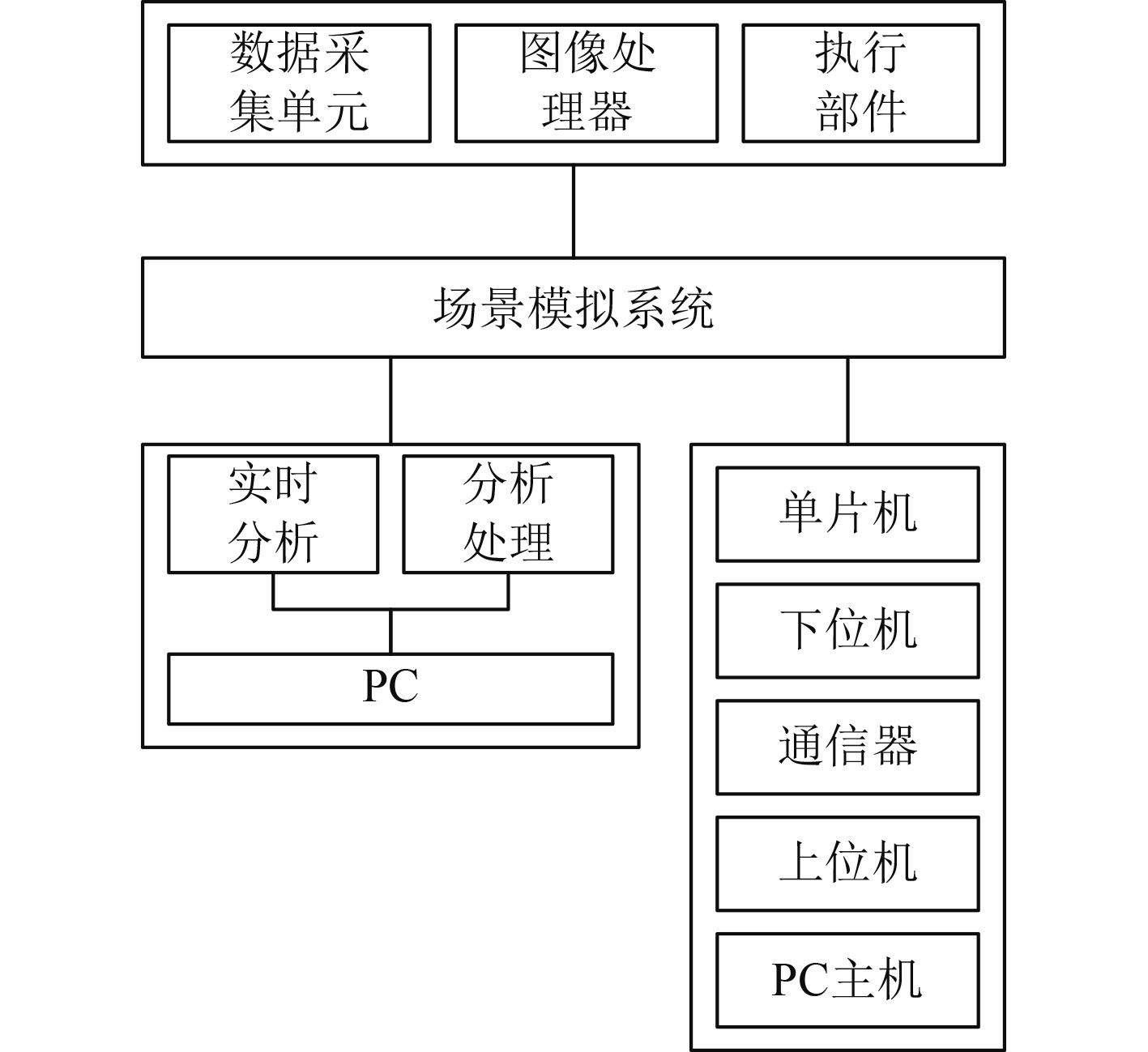图 1 交互式三维场景结构 Fig. 1 Interactive 3D scene structure
1.3 结果渲染输出系统设计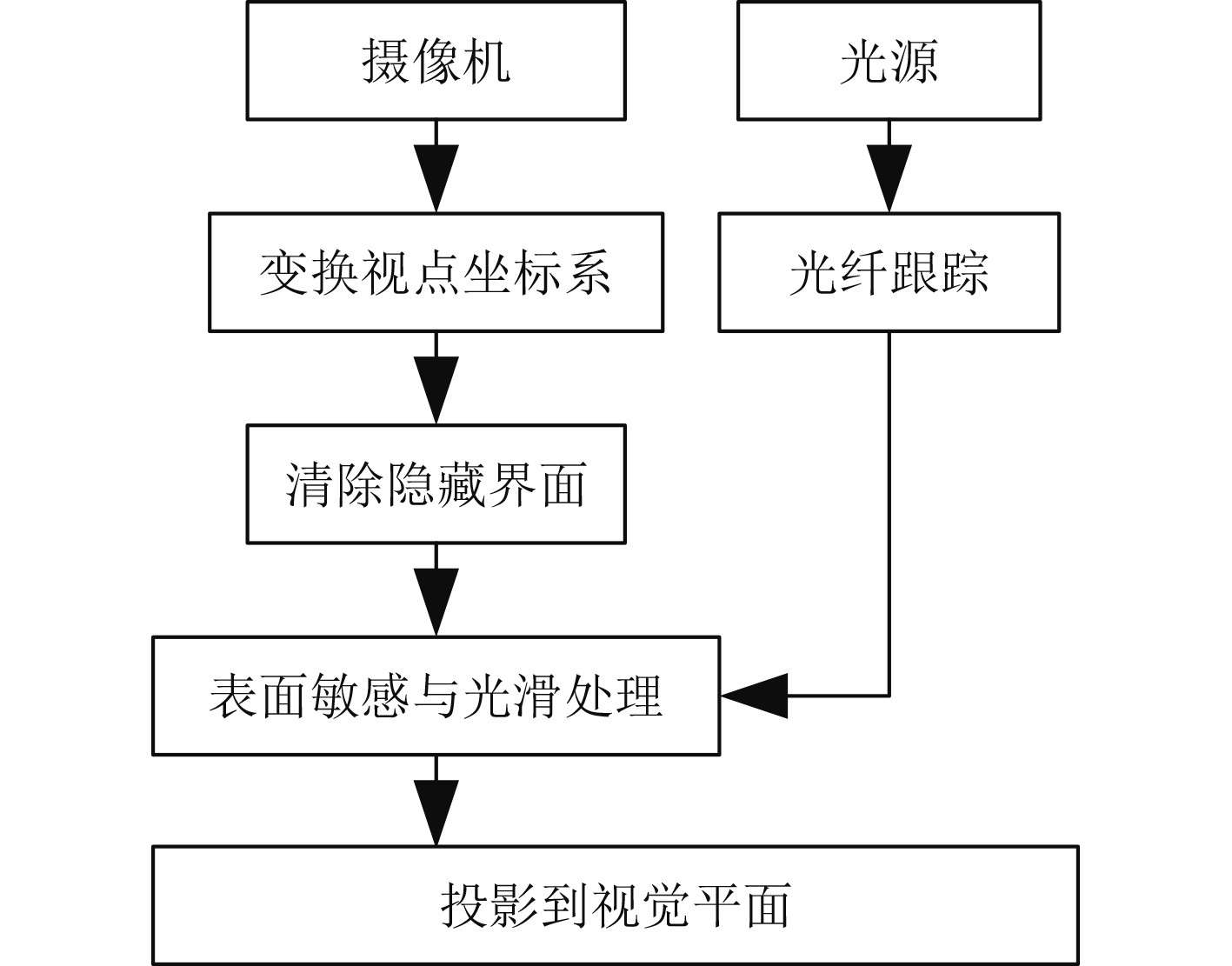图 2 结果渲染输出系统 Fig. 2 Result rendering output system
2 舰船通信移动终端UI大数据交互功能设计 2.1 三维动画功能设计

 $\iota = \frac{1}{z}\sum\limits_k o (k)。$ (3)

 $\left[ {\begin{array}{*{20}{c}} {{x_a}} \\ {{y_a}} \\ {{z_a}} \end{array}} \right] = \left[ {\begin{array}{*{20}{c}} {{o_x}}&{\dot x}&u \\ 0&{{o_y}}&{{u_0}} \\ 0&0&1 \end{array}} \right]。$ (4)

 $I\left(u\text{，}{u}_{0}\right)=\phi \sum _{k}o}(k)$ (5)

 $\left\{ \begin{gathered} x(a) = \sum\limits_{b = 0}^L I (a,b)，\\ y(a) = \sum\limits_{a = 0}^H I (a,b) 。\\ \end{gathered} \right.$ (6)

2.2 界面大数据交互功能设计

 $\left[ {\begin{array}{*{20}{c}} {x'}{y'}{z'}1 \end{array}} \right] = \left[ {\begin{array}{*{20}{c}} {{x_1} + {\gamma _{{x_1}}}}{{y_1} + {\gamma _{{y_1}}}}{{z_1} + {\gamma _{{z_1}}}}1 \end{array}} \right] 。$ (7)

 $\left[ {\begin{array}{*{20}{c}} {x''}{y''}{z''}1 \end{array}} \right] = \left[ {\begin{array}{*{20}{c}} {{x_1} \cdot \sin \theta }{{y_1} \cdot \cos \theta }{{z_1}}1 \end{array}} \right]。$ (8)

2.3 抗干扰功能设计

 $B = {f_e} + 2{l_{\min }}。$ (9)

 ${M_{\text{T}}} = \frac{{v\sqrt {{\varepsilon _r}} }}{{4{f_e}h}}，$ (10)

3 仿真实验 3.1 舰船操控台实验环境

3.2 移动终端数据检测实验装置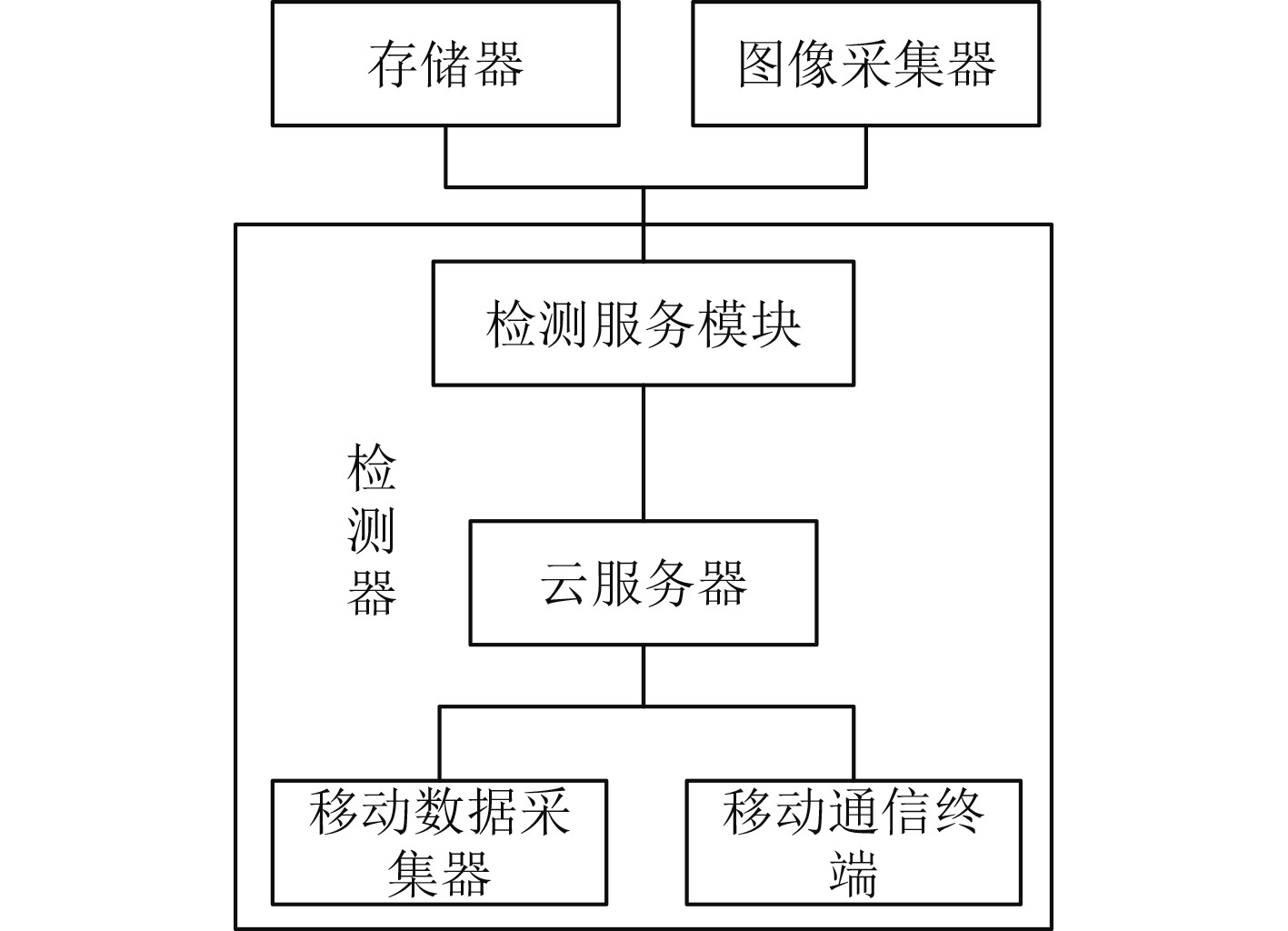图 3 移动终端数据检测实验装置 Fig. 3 Mobile terminal data detection experimental device
3.3 天线方向性图UI显示结果分析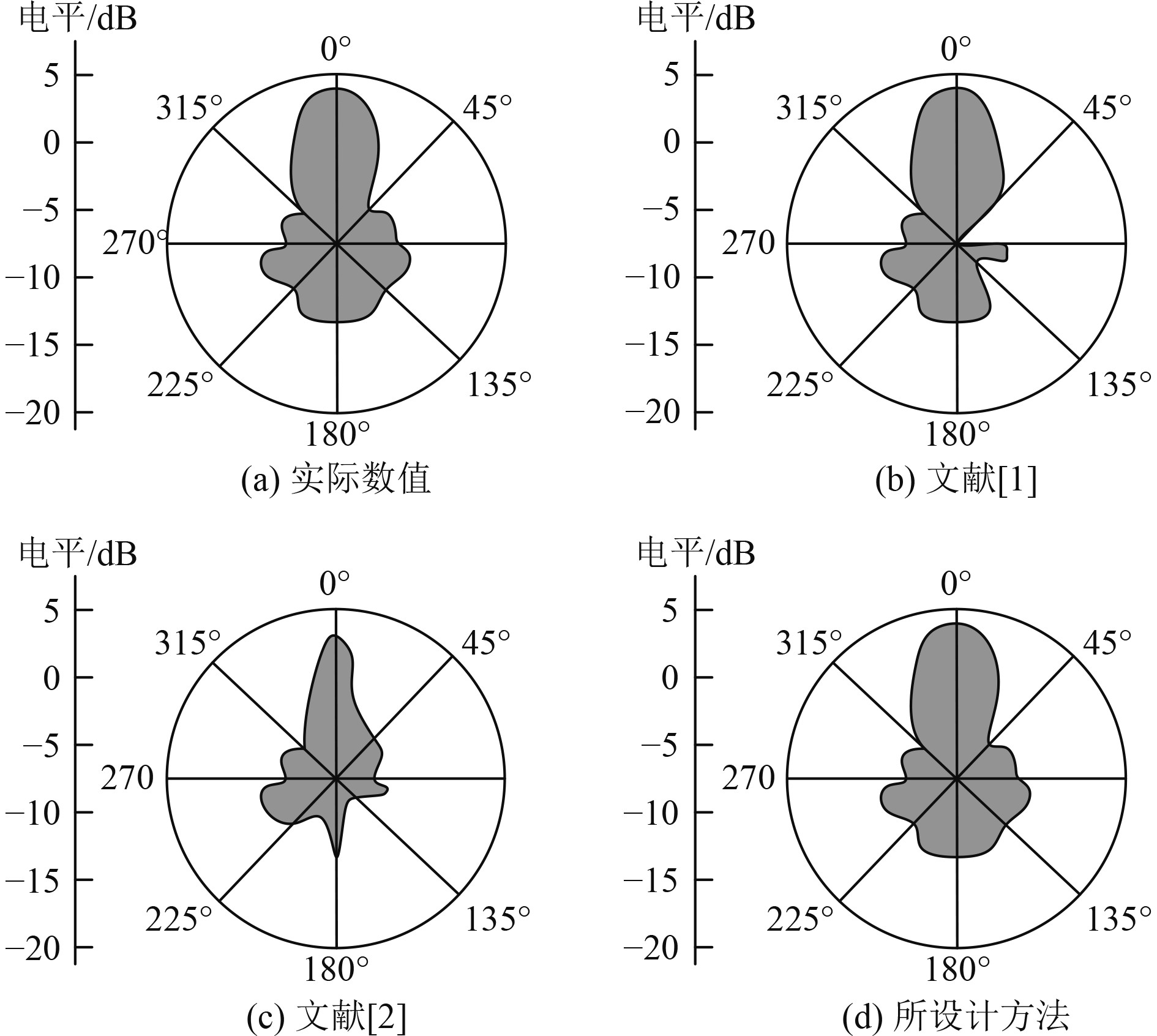图 4 不同方法天线方向性图UI显示结果对比分析 Fig. 4 Comparative analysis of the UI display results of antenna patterns of different methods
3.4 移动终端UI像素密度数值分析

 $n{\text{ = }}{f_p} \cdot \left( {L \times H} \right)。$ (11)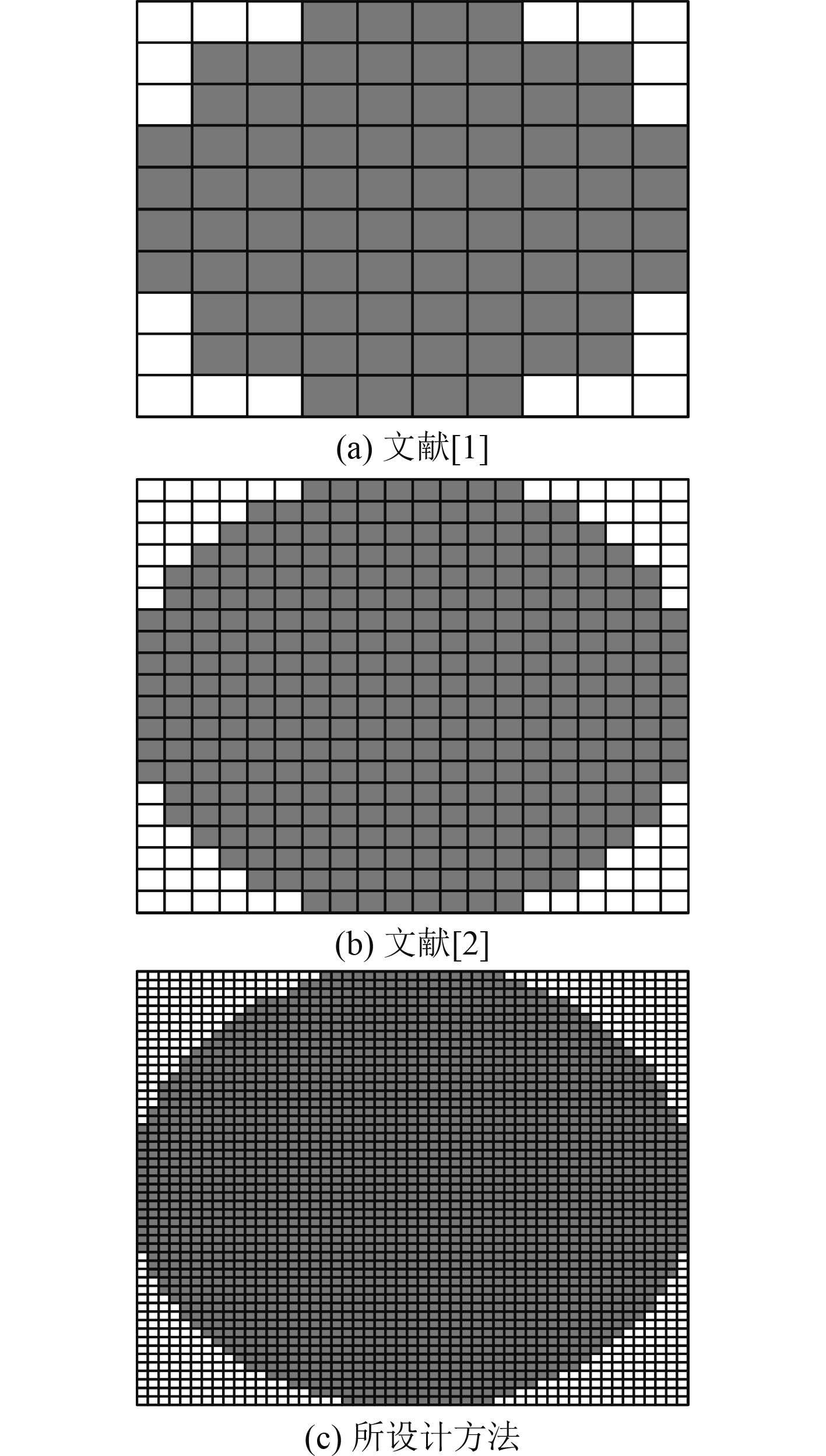图 5 不同方法像素密度对比分析 Fig. 5 Comparative analysis of pixel density of different methods
4 结　语

  翟延忠, 翟宝蓉, 马强, 等. 基于STM32的人机交互终端的设计[J]. 仪表技术与传感器, 2020(2): 105-109. DOI:10.3969/j.issn.1002-1841.2020.02.024  王兴涛, 赵训威, 孙孝波, 等. 230MHz电力无线专网通信终端设计与应用[J]. 电讯技术, 2021, 61(8): 999-1004. DOI:10.3969/j.issn.1001-893x.2021.08.013  王云棣, 王兴涛, 吴庆, 等. 1.8GHz电力无线专网远程通信终端设计[J]. 电子技术应用, 2020, 46(5): 88-92.  童向杰, 谢凤玲. 一种移动终端中提高卡接口可靠性的策略[J]. 电子器件, 2020, 43(4): 922-927. DOI:10.3969/j.issn.1005-9490.2020.04.041  张磊, 吴颖, 杨建常. 基于大数据挖掘的电力通信信号异常数据提取方法[J]. 科技通报, 2022, 38(6): 35-38+44. DOI:10.13774/j.cnki.kjtb.2022.06.006  徐林, 蒋祖运, 杨乾远, 等. 移动激光通信设备伺服稳定系统动态性能优化设计[J]. 光通信技术, 2020, 44(8): 38-42.  陈文, 向罗勇. 基于移动网络的电力移动终端安全通信探究[J]. 电视技术, 2022, 46(1): 41-43. DOI:10.16280/j.videoe.2022.01.011  刘为, 王琳, 温文坤, 等. 卫星移动通信地面终端单跳直通技术方案[J]. 中国电子科学研究院学报, 2021, 16(4): 374-385. DOI:10.3969/j.issn.1673-5692.2021.04.012